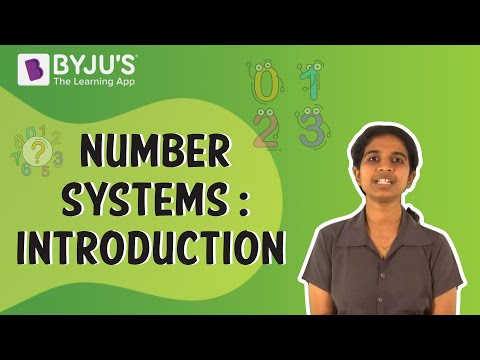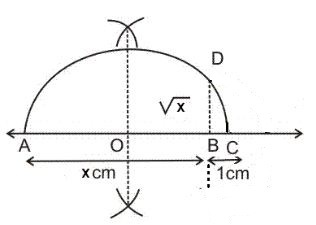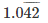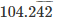# Number System Class 9 Notes - Chapter 1

## Introduction to Number Systems

### Numbers

Number: Arithmetical value representing a particular quantity. The various types of numbers are Natural Numbers, Whole Numbers, Integers, Rational Numbers, Irrational Numbers, Real Numbers etc.

To know more about Numbers, visit here.

### Natural Numbers

Natural numbers(N) are positive numbers i.e. 1, 2, 3 ..and so on.

To know more about Natural Numbers, visit here.

### Whole Numbers

Whole numbers (W) are 0, 1, 2,..and so on. Whole numbers are all Natural Numbers including ‘0’. Whole numbers do not include any fractions, negative numbers or decimals.
To know more about Whole Numbers, visit here.To know more about Number System, visit here.

### Integers

Integers are the numbers that includes whole numbers along with the negative numbers.

To know more about Integers, visit here.

### Rational Numbers

A number ‘r’ is called a rational number if it can be written in the form p/q, where p and q are integers and q ≠ 0.

To know more about Rational Numbers, visit here.

### Irrational Numbers

Any number that cannot be expressed in the form of p/q, where p and q are integers and q≠0, is an irrational number. Examples: √2, 1.010024563…, e, π

To know more about Irrational Numbers, visit here.

### Real Numbers

Any number which can be represented on the number line is a Real Number(R). It includes both rational and irrational numbers. Every point on the number line represents a unique real number.

To know more about Real Numbers, visit here.

## Irrational Numbers

### Representation of Irrational numbers on the Number line

Let √x be an irrational number. To represent it on the number line we will follow the following steps:

• Take any point A. Draw a line AB = x units.
• Extend AB to point C such that BC = 1 unit.
• Find out the mid-point of AC and name it ‘O’. With ‘O’ as the centre draw a semi-circle with radius OC.
• Draw a straight line from B which is perpendicular to AC, such that it intersects the semi-circle at point D.

Length of BD=√x.• With BD as the radius and origin as the centre, cut the positive side of the number line to get √x.

## Identities for Irrational Numbers

Arithmetic operations between:

• rational and irrational will give an irrational number.
• irrational and irrational will give a rational or irrational number.

Example : 2 × √3 = 2√3 i.e. irrational. √3 × √3 = 3 which is rational.

### Identities for irrational numbers

If a and b are real numbers then:

• √ab = √a√b
• √ab = √a√b
• (√a+√b) (√a-√b) = a – b
• (a+√b)(a−√b) = a²−b
• (√a+√b)2 = a+2√(ab)+b

### Rationalisation

Rationalisation is converting an irrational number into a rational number. Suppose if we have to rationalise 1/√a.
1/√a × 1/√a = 1/a

Rationalisation of 1/√a+b:

(1/√a+b) × (1/√a−b) = (1/a−b²)

### Laws of Exponents for Real Numbers

If a, b, m and n are real numbers then:

• a× an= am+n
• (am) = amn
• am/a= am−n
• ambm=(ab)m

Here, a and b are the bases and m and n are exponents.

### Exponential representation of irrational numbers

If a > 0 and n is a positive integer, then: n√a=a1n Let a > 0 be a real number and p and q be rational numbers, then:

• ap × aq = ap + q
• (ap)= apq
• ap/ aq= ap−q
• apb= (ab)p

## Decimal Representation of Rational Numbers

### Decimal expansion of Rational and Irrational Numbers

The decimal expansion of a rational number is either terminating or non- terminating and recurring.

Example: 1/2 = 0.5 , 1/3 = 3.33…….
The decimal expansion of an irrational number is non terminating and non-recurring.
Examples: √2 = 1.41421356..

### Expressing Decimals as rational numbers

Case 1 – Terminating Decimals

Example – 0.625

Let x=0.625

If the number of digits after the decimal point is y, then multiply and divide the number by 10y.

So, x = 0.625 × 1000/1000 = 625/1000 Then, reduce the obtained fraction to its simplest form.

Hence, x = 5/8

Case 2: Recurring Decimals

If the number is non-terminating and recurring, then we will follow the following steps to convert it into a rational number:

Example –Step 1. Let x =(1)

Step 2. Multiply the first equation with 10y, where y is the number of digits that are recurring.

• Thus, 100x =(2)Steps 3. Subtract equation 1 from equation 2.On subtracting equation 1 from 2, we get99x = 103.2x=103.2/99 = 1032/990

Which is the required rational number.

Reduce the obtained rational number to its simplest form Thus,

x=172/165

To know more about Decimal Representation of Rational Numbers, visit here.